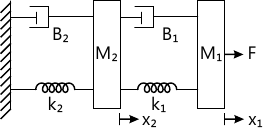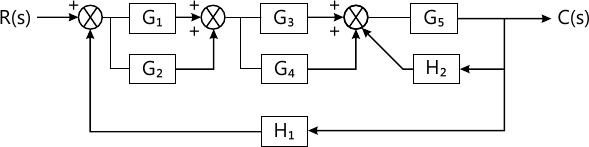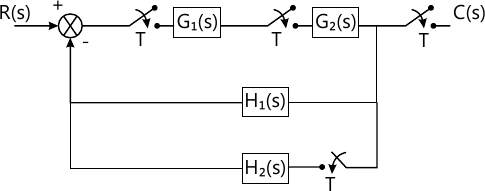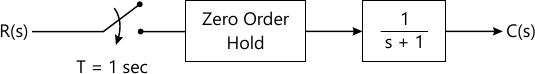Total marks: --
Total time: --
INSTRUCTIONS
(1) Assume appropriate data and state your reasons
(2) Marks are given to the right of every question
(3) Draw neat diagrams wherever necessary

Answer any one question from Q1 and Q2
1 (a) Give the various terminology of electrical system and its analogous quantities based on force-current analogy.
6 M
1 (b) Write the differential equations of system shown in Fig. 1. Also find $\dfrac {X_1 (s)} {F(s)}$6 M

2 (a) Obtain transfer function of the system shown in Fig. 2:6 M
2 (b) The open loop transfer function of unity feedback system is $G(s) = \dfrac {k_1} {s(\tau s +1)} \ with \ k, \ \tau>0$ with a given of k1, the peak overshoot was found to be 80%. if the overshoot is decreased up to 20% by new gain k2 find k2 in terms of k1.
6 M

Answer any one question from Q3 and Q4
3 (a) Using Routh's criteria, comment on the stability if characteristic equation is:
S5+2s4=3s3+8s2+s+1=0
4 M
3 (b) Draw the Bode plot and obtain gain margin, phase margin, gain crossover frequency and phase crossover frequency if: $G(s)\cdot H(s)= \dfrac {50,000 (s+10)}{s(s+1)(s+500)} 8 M 4 (a) \[ if\ G(s) H(s) = \dfrac {k}{s(s+1)(s+10)}$ sketch the complete Root locus and comment on the stability.
8 M
4 (b) $If \ G(s) \ H(s)= \dfrac {1}{s(s+1)}$ Find Resonance peak and Resonance frequency,
4 M

Answer any one question from Q5 and Q6
5 (a) Obtain transfer function of state model if: $A=\begin{bmatrix} 0 &1 &0 \\0 &0 &1 \\-6 &-11 &-6 \end{bmatrix}, \ B=\begin{bmatrix} 0\\0 \\1 \end{bmatrix}, \ C=\begin{bmatrix} 1 &0 &0 \end{bmatrix}, \ D=$
6 M
5 (b) Find controllability and observability of the state model: $A=\begin{bmatrix} 1 &0 &1 \\0 &1 &1 \\1 & 1 &1 \end{bmatrix}, \ B=\begin{bmatrix} 1\\1 \\1 \end{bmatrix}, \ C=\begin{bmatrix} 1 &1 &1 \end{bmatrix}, \ D=$
7 M

6 (a) Obtain state transition matrix if: $x= \begin{bmatrix} 0 &-1 \\-11 &-12 \end{bmatrix} x($ using Laplace transformation.
6 M
6 (b) With the help of general equation, explain concept of controllable canonical and observable canonical form of state space.
7 M

Answer any one question from Q7 and Q8
7 (a) Enlist various terms in PID controller with sketch of output of P, PI, PD and PID controller for step input.
6 M
7 (b) Find pulse transfer function of Fig. 3.7 M

8 (a) Explain any one application of PLC with ladder diagram.
6 M
8 (b) Obtain unit step response of the system shown in Fig. 47 M

More question papers from Control Systems